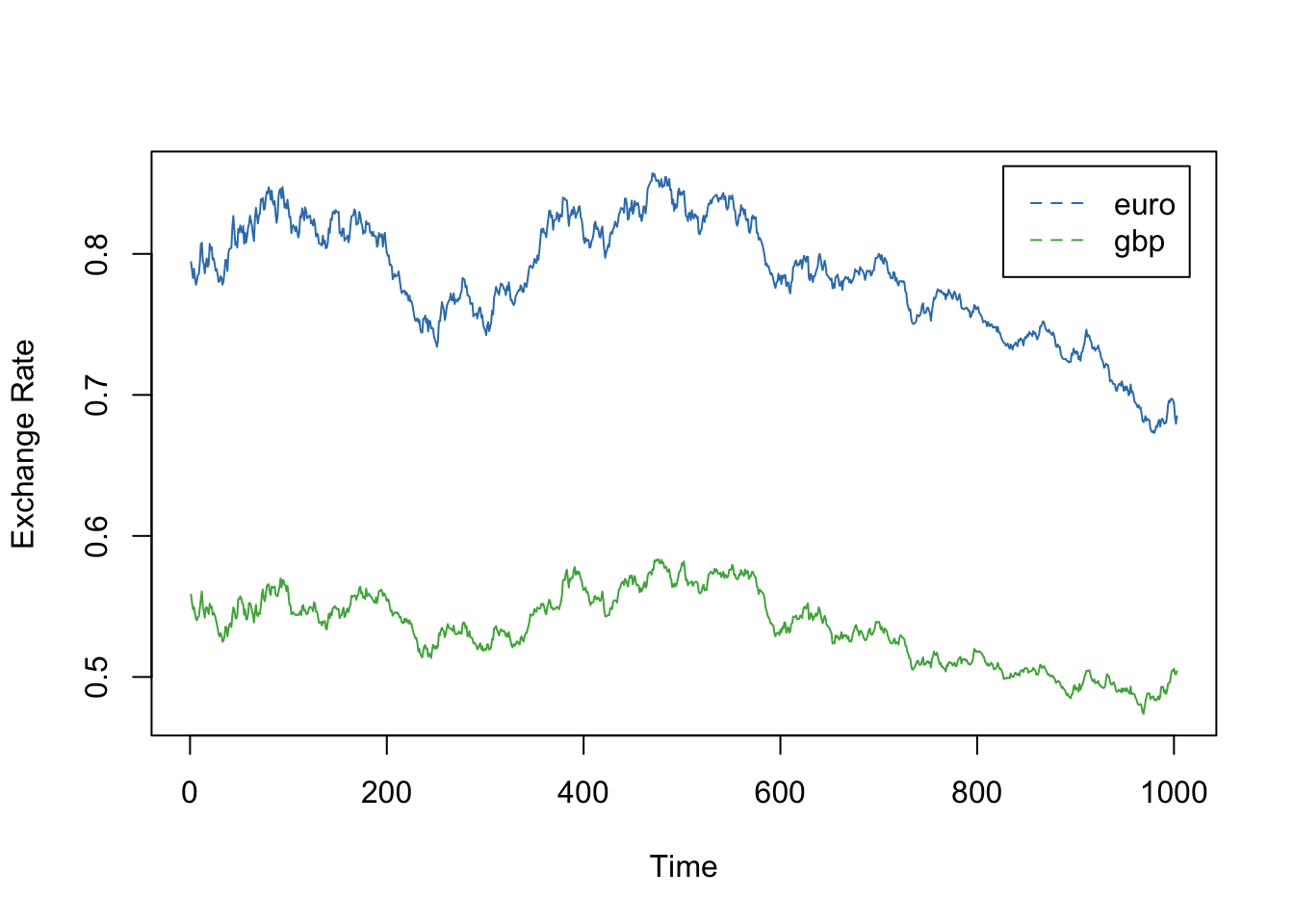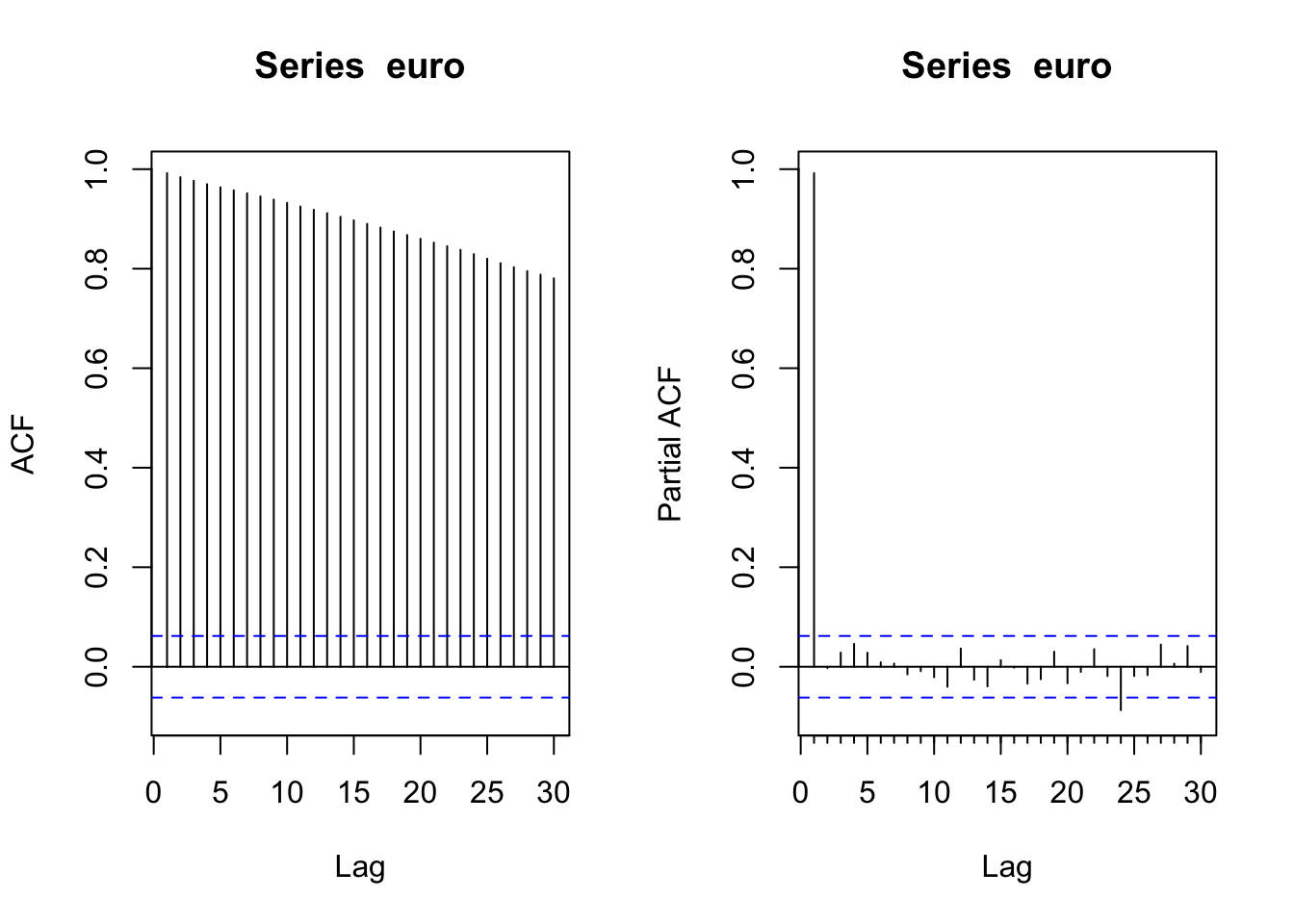## Preamble

This lecture is based on Cowpertwait & Metcalfe, Introductory Time Series with R, Chapter 11.

library("forecast")
library("tseries")

## Exchange Rate Data

# Daily exchange rates for UK pounds and the Euro,
# from January 2004 to December 2007, per US dollar.
#
n <- nrow(xrates)
time <- 1:n

euro <- xrates$EU gbp <- xrates$UK

## Time Series Plots

ylim <- range(euro, gbp)
plot(time, euro, ylim=ylim, type="l", col=2, ylab="Exchange Rate", xlab="Time")
lines(time, gbp, col=3)
legend("topright", inset=0.025, legend=c("euro", "gbp"), col=c(2, 3), lty=2)## ACFs, PACFs for Euro

Euro

par(mfrow=c(1,2))
Acf(euro)
Pacf(euro)Diff. Euro

par(mfrow=c(1,2))
Acf(diff(euro))
Pacf(diff(euro))